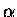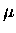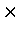Next: Electron energy spectrum Up: Corrections to in the Previous: Corrections to in the

### Charged particles from muon capture

Muon capture on a proton,

 (86)

is an elementary process (at least at the nucleon level), but when the muon is captured on a nucleus the process is rather complex. Muon capture in a heavy nucleus often leads to the emission of particles, normally neutrons but sometimes charged particles. These have the same time constants as the decay electrons, hence if detected in either the scintillator pairs or the telescope, they would bias the determination of the stopping fraction from the amplitude ratio.

The detection of charged particles would modify the branching ratio of muon decay versus total muon disappearance (Eq. C.4) into an effective branching ratio ,

 (87)

where Wmp are fractional probabilities per muon capture for emission of m charged particles. Alternatively, the effect can be incorporated as the efficiency for the electron detection in Eq. 6.5

 = (88) = (89)

with defined as a correction factor.

Since the ratio is for the case of k = gold, a relatively small fraction of charged particle emission can affect the stopping fraction determination, hence this effect needs to be considered in some detail. The charged particle emission following muon capture has been traditionally less studied compared to neutral emission, but there is some recent interest in relation to nucleon pairing in nuclei. A few reviews exist on this subject [219,220,221]. Following muon capture, the emission of neutrons (and gammas) dominates over that of charged particles ( etc.), as the latter requires a multi-step reaction or capture on correlated nucleons, since the process (6.7) only gives outgoing neutrons. The total probability of the charged particle emission decreases with increasing Z of the nucleus, due to the increasing Coulomb barrier [219,220], but there is some evidence from recent measurements that the yield of the highest energy protons is independent of Z .

Table 6.9 lists measured and calculated probabilities for charged particle emission reactions ( ), where xis the neutron multiplicity, and includes deuterons and tritons as well as protons. Column (a) is the experimental lower limit from observed channels, while (b) shows the estimated total inclusive probabilities derived from measured channels, corrected by an approximate regularity among various channels. Column (c) gives calculations by Lifshitz and Singer, and (d) those by Wyttenbach et al.

Table 6.9: Measured and calculated fractional probabilities (per captured muon) for inclusive charged particle emission reactions ( ). (a) experimental lower limit for observed channels, (b) estimated total inclusive probabilities derived from (a) using approximate ratio of various channel, (c) and (d) theoretical calculations. (a), (b) and (c) are from Ref. , and (d) from Ref. .
 Nucleus Probability per muon capture (%) (a) (b) (c) (d) 6329Cu >1.70.3 3.6 2.5 18173Ta >0.070.01 0.30 0.28 0.29 20882Pb >0.300.08 0.41 0.11 0.22

We ignore the charged particle multiplicity in the following analysis, i.e., we let . Note that emission ofparticles is suppressed by at least several times . Assuming % for gold, we obtain from Eq. 6.9, which in turn gives an upward correction to the apparent hydrogen stopping fraction . The magnitude of the correction varies from 0 to 14% (relative) as a decreasing function of , but for %, the corrected value will be , a relative shift of 10%.

For copper which is also present in parts of the target system, the emission probability is nearly an order of magnitude higher than gold (Table 6.9). However, the fit to decay electron data with three exponential functions, two of which were fixed to the lifetimes corresponding to Au and Cu measured by Suzuki, Measday and Roalsvig , indicated that the apparent Cu component cannot be more than 10% (in most cases less than a few percent) of gold. Furthermore, the capture rate for copper is less than half that of gold, while the Huff factor for copper is larger. All this suggests that the relative contribution of charged particles from copper is significantly smaller than that from gold and thus is neglected.

Nevertheless, the estimate of 14% effect on is a worst possible case of detecting all the charged particles produced. In reality, the emitted particles can be ranged out in the walls and other materials, hence the effect is expected to be smaller. This of course depends on the energy spectrum of the emitted charged particles, which we discuss in the following.

Table 6.10, based on a compilation by Cummings , lists previously measured yields of high energy protons following muon capture, integrated above a threshold energy Eth.

Table 6.10: Integrated yields of proton emission following muon capture, based on the compilation in Ref. . Eth is the energy threshold above which the yield is integrated. E 0 is derived from a fit of the energy spectrum to the form .
 Nucleus Authors Eth E 0 (MeV) (10-4) (MeV) S Baladin et al. (1979)  40.5 2.070.15 6.40.1 Ca Martoff et al. (1991)  40 2.320.26 8.01.5 Cu Budyashov et al. (1971)  42.5 3.81.4 13.11.6 Cu Krane et al. (1979)  40 19.61.2 8.30.5 Y Cummings (1991)  40 0.720.07 7.21.0 Pb Krane et al. (1979)  40 1.710.28 9.91.1

According to Cummings , some of the older results by Budyashov et al. and by Krane et al. may be unreliable, and excluding those would suggest high energy proton yields of 1-2 , independent of the atomic number.

For the En2 counter located downstream of the target, there is substantial material between the gold target support and the detector, including 1.6 mm gold-plated copper, 2.5 mm stainless steel and 3 mm Plexiglas. According to the ICRU range table , the copper and stainless steel alone can stop protons up to MeV. Therefore, the effect of charged particles should be less than of the order of 10-3, which is completely negligible for the SH determination.

On the other hand, the amount of material between the gold and electron counters on the sides of the target (Ege, En1) is considerably less, in particular, for the paths which go through the silicon detector. A rough estimate based on the range table  suggests that protons of energies above 20 to 25 MeV may reach the second scintillator E2ge (providing a coincidence of the scintillator pair) after going through the silicon detector (300m), a copper thermal shield foil (13m), a stainless steel window (76m), and the first scintillator E1ge (3.2 mm) with three layers of mylar wrappings (3285m) (recall that Ege = E1ge E2ge).

Assuming the proton spectrum from the gold is similar to that from yttrium given by Cummings  and using the partial yield above 40 MeV and the exponential decay rate in Table 6.10, the yield above 20 MeV, can be derived to be . This would give (see Eq. 6.9), which in turn would give a relative 6% correction, if %, to 26.5% from Eq. 6.5.

However, if the charged particle did not go through a Si detector, but hit the Cu collimator around the active Si area, it would be less likely to reach the scintillators. The relative acceptance of Ege (or En1) compared to that of Si detector, which was assumed to be unity above, is actually less than a half, hence the effect of charged particles is expected to be smaller than the above, and of a few percent level for the side counters Ege and En1.Next: Electron energy spectrum Up: Corrections to in the Previous: Corrections to in the